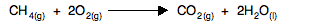CALCULATING ENTROPY CHANGES

```
```

This page looks at how you can calculate entropy changes during reactions from given values of entropy for each of the substances taking part.

Note:  If you haven't already read the page about introducing entropy, you should do so before you go on.

This page deals only with entropy changes to the system. Entropy change to the surroundings and the total entropy change are dealt with on another page. If your syllabus doesn't mention all these different sorts, just ignore this comment.

Standard entropies, S°

Entropy is given the symbol S, and standard entropy (measured at 298 K and a pressure of 1 bar) is given the symbol S°. You might find the pressure quoted as 1 atmosphere rather than 1 bar in less recent sources. Don't worry about it - they are nearly the same. 1 bar is 100 kPa; 1 atmosphere is 101.325 kPa. Use whatever units the examiners give you.

The units of entropy are J K-1mol-1. The thing you must be most careful about is the fact that entropy is measured in joules, not kilojoules, unlike most of the other energy terms you will have come across.

In an exam, you will be given values for all the standard entropies you need.

```
```

Entropy changes, ΔS°

Working out entropy changes for a reaction is very easy.

You add up the entropies for everything you end up with, and take away the entropies of everything you started with.

 Change in entropy = what you end up with - what you started with
```
```

Or if you like things mathematical:

ΔS° = ΣS°(products) - ΣS°(reactants)

Where Σ (sigma) simply means "the sum of".

```
```

In the introductory page we looked at the following reaction and worked out that there would be a decrease in entropy. Let's now do the calculation.CH4(g) O2(g) CO2(g) H2O(l) S° (J K-1mol-1) 186 205 214 69.9
```
```

You started with 1 mole of methane and 2 moles of oxygen.

Total starting entropy = 186 + 2(205) = 596 J K-1mol-1

You ended up with 1 mole of carbon dioxide and two moles of liquid water.

Total entropy at the end = 214 + 2(69.9) = 353.8 J K-1mol-1

Entropy change = what you end up with - what you started with.

Entropy change = 353.8 - 596 = -242.2 J K-1mol-1

Notice that it is a negative value. The entropy has decreased - as we predicted it would in the earlier page. That's because there is a decrease in the total number of gas molecules present.

```
```

And that is all there is to it! You will, of course, need to practise doing this until you are completely confident, but you will need to find your own examples. There are lots in my calculations book if you have a copy. You will find examples on pages 260 to the top of page 262, and in problems 15 and 16 in the end-of-chapter questions. For the purposes of this page, you can ignore any reference to the word "system".

```
```
 Questions to test your understanding Because this is all covered in detail in my calculations book I shan't be setting any questions throughout this section on entropy and free energy
```
```

Where would you like to go now?

To the entropy and free energy menu . . .

To the Physical Chemistry menu . . .

To Main Menu . . .

```
```

© Jim Clark 2017